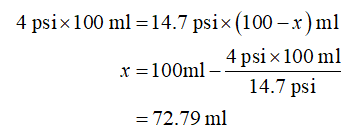# Use your own total amount of Freon and size of container.At 60°F, freon-12 (dichlorodifluoromethane) has a vapor pressure of 4 pounds per square inch (1.0 atm = 14.7 psi). If I opened a pressure vessel containing liquid freon-12 and poured it into an open beaker in the lab how much liquid freon would remain when equilibrium is established? Explain.

Question
13 views

Use your own total amount of Freon and size of container.

At 60°F, freon-12 (dichlorodifluoromethane) has a vapor pressure of 4 pounds per square inch (1.0 atm = 14.7 psi). If I opened a pressure vessel containing liquid freon-12 and poured it into an open beaker in the lab how much liquid freon would remain when equilibrium is established? Explain.

check_circle

Step 1

The vapor pressure of the liquid depends upon the external pressure and when the pressure of the system is increases the amount of vapor decreases due to condensation process. In the given question the pressure is increased from 4 psi to 14.7 psi (the atmospheric pressure).

Step 2

Consider the volume of vapor at 4psi is 100 ml and x is the volume of vapor which is converted into the liquid through the condensation process. the volume of the vapor that converted into liquid is 72.79 ml....

### Want to see the full answer?

See Solution

#### Want to see this answer and more?

Solutions are written by subject experts who are available 24/7. Questions are typically answered within 1 hour.*

See Solution
*Response times may vary by subject and question.
Tagged in